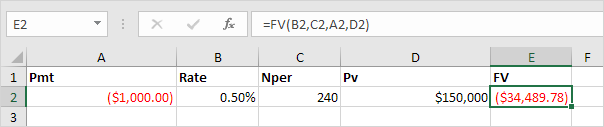## Excel Office

Excel How Tos, Tutorials, Tips & Tricks, Shortcuts

# PMT, RATE, NPER, PV and FV Financial Functions in Excel

Learn all about Excel’s PMT, RATE, NPER, PV and FV

To illustrate Excel’s most popular financial functions, we consider a loan with monthly payments, an annual interest rate of 6%, a 20-year duration, a present value of \$150,000 (amount borrowed) and a future value of 0 (that’s what you hope to achieve when you pay off a loan).

We make monthly payments, so we use 6%/12 = 0.5% for Rate and 20*12 = 240 for Nper (total number of periods). If we make annual payments on the same loan, we use 6% for Rate and 20 for Nper.

### PMT:

Calculates the payment for a loan based on constant payments and a constant interest rate.Syntax for PMT:

PMT(rate, nper, pv, [fv], [type])

Select cell A2 and insert the PMT function.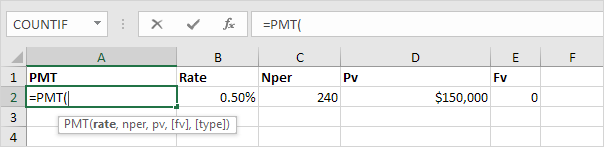Note: the last two arguments are optional. For loans, Fv can be omitted (the future value of a loan equals 0, however, it’s included here for clarification). If Type is omitted, it is assumed that payments are due at the end of the period.

Worked Example:   PMT function: Description, Usage, Syntax, Examples and Explanation

Result. The monthly payment equals \$1,074.65.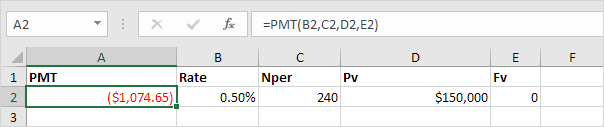Tip: when working with financial functions in Excel, always ask yourself the question, am I making a payment (negative) or am I receiving money (positive)? We pay off a loan of \$150,000 (positive, we received that amount) and we make monthly payments of \$1,074.65 (negative, we pay). Visit our page about the PMT function for many more examples.

### RATE:

Returns the interest rate per period of an annuity.Syntax for RATE:

RATE(nper, pmt, pv, [fv], [type], [guess])

If Rate is the only unknown variable, we can use the RATE function to calculate the interest rate.

Worked Example:   Example of payment for annuity in Excel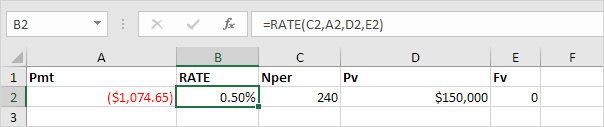### NPER:

Returns the number of periods for an investment based on periodic, constant payments and a constant interest rate.Syntax for NPER:

NPER(rate,pmt,pv,[fv],[type])

Or the NPER function. If we make monthly payments of \$1,074.65 on a 20-year loan, with an annual interest rate of 6%, it takes 240 months to pay off this loan.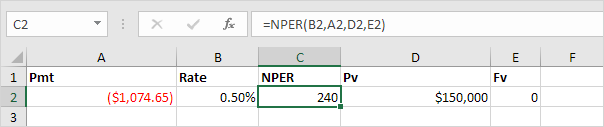We already knew this, but we can change the monthly payment now to see how this affects the total number of periods.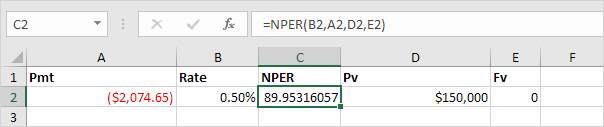Conclusion: if we make monthly payments of \$2,074.65, it takes less than 90 months to pay off this loan.

### PV:

Returns the present value of an investment.

Worked Example:   Calculate periods for annuity in Excel

Syntax for PV:

PV(rate, nper, pmt, [fv], [type])

Or the PV (Present Value) function. If we make monthly payments of \$1,074.65 on a 20-year loan, with an annual interest rate of 6%, how much can we borrow? You already know the answer.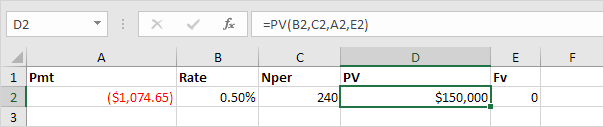### FV:

Returns the future value of an investment based on periodic, constant payments and a constant interest rate.

Syntax for FV:

FV(rate,nper,pmt,[pv],[type])

And we finish this chapter with the FV (Future Value) function. If we make monthly payments of \$1,074.65 on a 20-year loan, with an annual interest rate of 6%, do we pay off this loan? Yes.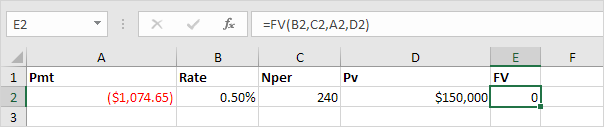But, if we make monthly payments of only \$1,000.00, we still have debt after 20 years.